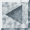CS831 Exam Description

The CS831 exam consists of approximately 5 questions worth approximately 15 marks each.

These questions relate to the lectures presented by the course instructor. They do NOT relate to material presented by graduate students. The best reference material is the lecture notes provided on the course website.

In broad form, the questions relate to the following topics:

1. Version Spaces
• you should be able to answer questions about the purpose, meaning, and use of a version space
• you should be able to construct a version space for a set of training instances
2. Decision Trees
• you should be able to answer questions about the purpose, meaning, and use of a decision tree
• you should be able to construct a decision tree for a set of training instances
• you are not required to know how to prune decision trees
3. Evaluation of Data Mining
• You should be able to answer questions about the purpose, meaning, and use of a confusion matrix, an ROC graph, a cumulative gains chart, and a lift chart.
• You should be able to draw a confusion matrix, an ROC graph, a cumulative gains chart, and a lift chart for sample data.
4. Data Cubes and Summarization
• you should be able to answer questions about the purpose, meaning, and use of data cubes and iceberg cubes.
• you should be able to answer questions about the representation of a data cube in m-dimensional format, in ordered sets format, and with included totals.
• you should be able to draw a data cube corresponding to sample data, including its appearance before and after summarization (rollup) and drill down.
• you should be able to draw the generalization space corresponding to concept hierarchies.
• you should be able to demonstrate how the APRIORI algorithm applies to iceberg cubes.
• you are not required to know how the TDC and BUC methods are used to compute iceberg cubes.
5. Itemsets and Association Rules
• you should be able to answer questions about the purpose, meaning, and use of itemsets, support, confidence, share, and association rules
• you should be able to determine the support and confidence for items and itemsets based on a set of transactions
• you should be able to determine frequent itemsets using the APRIORI algorithm
• you should be able to determine association rules between items/itemsets based on information about support, share, and confidence.
• you are not required to describe dynamic itemsets or the DIC algorithm.
6. Clustering
• you should be able to answer questions about the purpose, meaning, and use of distance functions, supervised learning, nonsupervised learning, and clustering algorithms
• you should be able to calculate the Euclidian and manhatten distances between tuples of m values.
• given a k-means clustering algorithm, an agglomerative hierarchical clustering algorithm, or a similar algorithm, you should be able to apply it to sample data
• you should be able to draw a dendogram (tree diagram) corresponding to the results of a hierarchical clustering algorithm